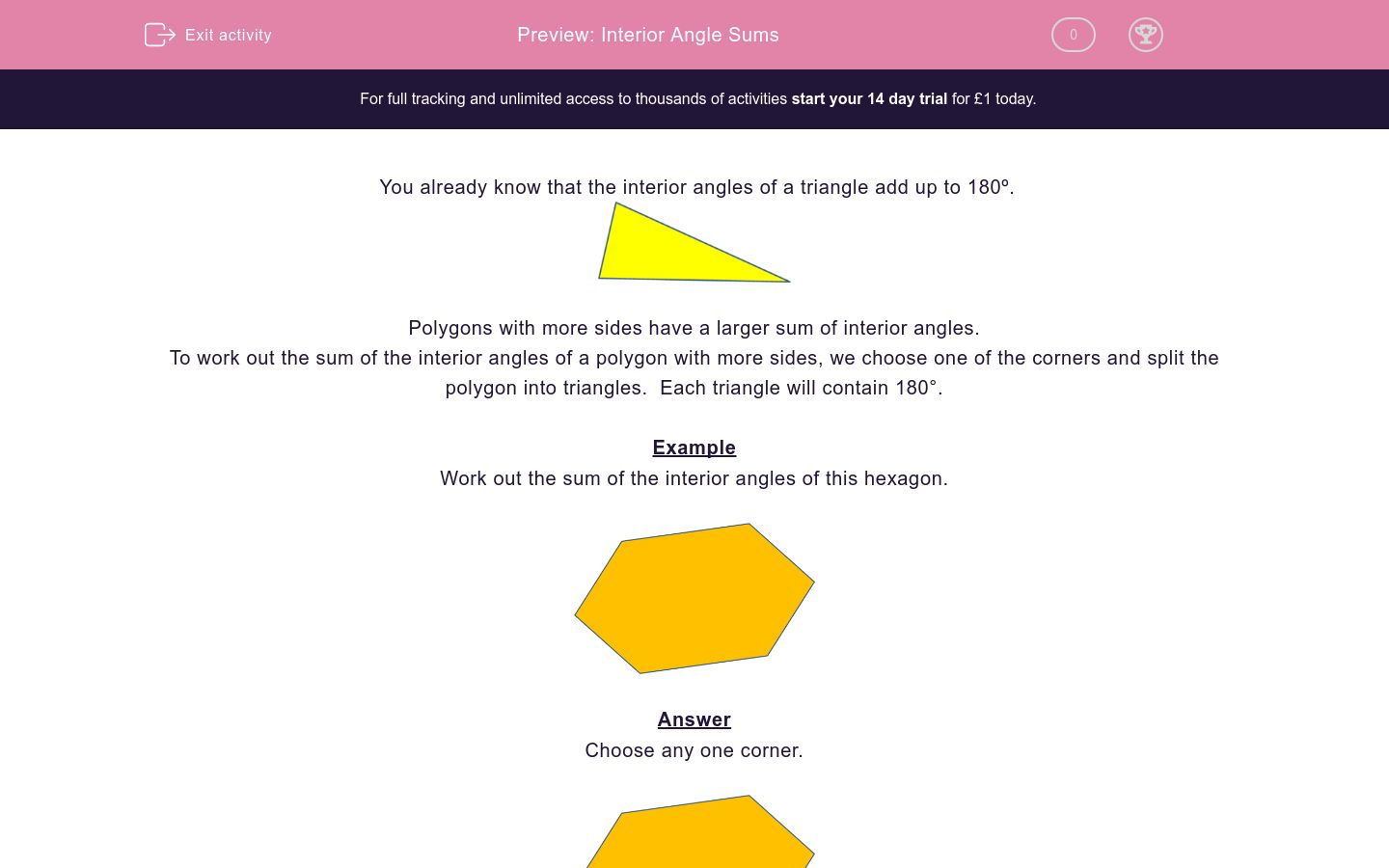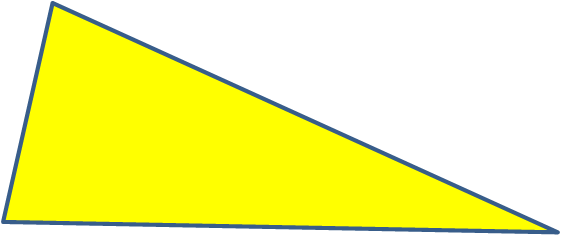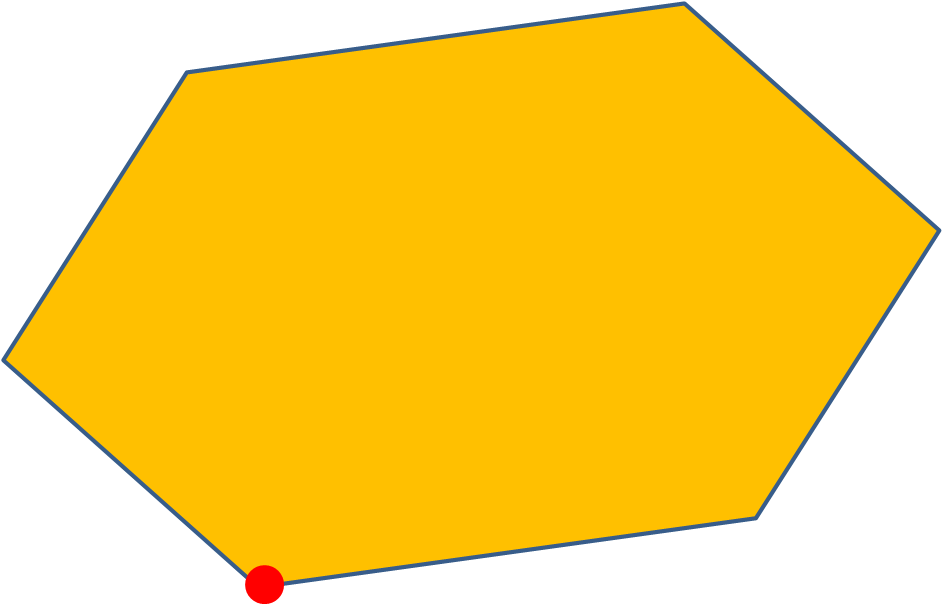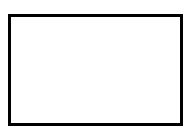# Interior Angle Sums

In this worksheet, students calculate the sum of the interior angles of the given polygons.Key stage:  KS 2

Curriculum topic:   Maths and Numerical Reasoning

Curriculum subtopic:   2D Shapes: Triangles, Quadrilaterals and Polygons

Difficulty level:### QUESTION 1 of 10

You already know that the interior angles of a triangle add up to 180º.Polygons with more sides have a larger sum of interior angles.

To work out the sum of the interior angles of a polygon with more sides, we choose one of the corners and split the polygon into triangles.  Each triangle will contain 180°.

Example

Work out the sum of the interior angles of this hexagon.Choose any one corner.Draw diagonals from the chosen corner to form triangles.There are 4 triangles inside the hexagon.

4 x 180° = 720°

The sum of the interior angles of a hexagon is 720°.

General Rule:

When you have a shape with n sides, the number of triangles it can be split into is n-2

Once you have the number of triangles, we can simply multiply that by 180° to find the internal angles.

For example: A 5 sided shape can be split into 3 triangles, if you then multiply 3 by 180 you get that there are 540° in a 5 sided shape.

Work out the sum of the interior angles of this rectangle in degrees.

(just write the number)Work out the sum of the interior angles of this heptagon in degrees.

(just write the number)Work out the sum of the interior angles of this pentagon in degrees.

(just write the number)Work out the sum of the interior angles of this irregular pentagon in degrees.

(just write the number)Work out the sum of the interior angles of this octagon in degrees.

(just write the number)What is the sum of the interior angles of any quadrilateral?

(just write the number)

What is the sum of the interior angles of any nonagon (9 sides)?

(just write the number)

What is the sum of the interior angles of any decagon ?

(just write the number)

What is the sum of the interior angles of any 12-sided polygon ?

(just write the number)

What is the sum of the interior angles of any 11-sided polygon ?

(just write the number)

• Question 1

Work out the sum of the interior angles of this rectangle in degrees.

(just write the number)360
EDDIE SAYS
This shape has 4 sides That means it can be split into 2 triangles 2 x 180 = 360°
• Question 2

Work out the sum of the interior angles of this heptagon in degrees.

(just write the number)900
EDDIE SAYS
This shape has 7 sides That means it can be split into 5 triangles 5 x 180 = 900°
• Question 3

Work out the sum of the interior angles of this pentagon in degrees.

(just write the number)540
EDDIE SAYS
This shape has 5 sides That means it can be split into 3 triangles 3 x 180 = 540°
• Question 4

Work out the sum of the interior angles of this irregular pentagon in degrees.

(just write the number)540
EDDIE SAYS
This shape has 5 sides That means it can be split into 3 triangles 3 x 180 = 540°
• Question 5

Work out the sum of the interior angles of this octagon in degrees.

(just write the number)1080
EDDIE SAYS
This shape has 8 sides That means it can be split into 6 triangles 6 x 180 = 1080°
• Question 6

What is the sum of the interior angles of any quadrilateral?

(just write the number)

360
EDDIE SAYS
These shapes have 4 sides That means they can be split into 2 triangles 2 x 180 = 360°
• Question 7

What is the sum of the interior angles of any nonagon (9 sides)?

(just write the number)

1260
EDDIE SAYS
These shapes have 9 sides That means they can be split into 7 triangles 7 x 180 = 1260°
• Question 8

What is the sum of the interior angles of any decagon ?

(just write the number)

1440
EDDIE SAYS
These shapes have 10 sides That means they can be split into 8 triangles 8 x 180 = 1440°
• Question 9

What is the sum of the interior angles of any 12-sided polygon ?

(just write the number)

1800
EDDIE SAYS
These shapes have 12 sides That means they can be split into 10 triangles 10 x 180 = 1800°
• Question 10

What is the sum of the interior angles of any 11-sided polygon ?

(just write the number)

1620
EDDIE SAYS
These shapes have 11 sides That means they can be split into 9 triangles 9 x 180 = 1620°
---- OR ----

Sign up for a £1 trial so you can track and measure your child's progress on this activity.

### What is EdPlace?

We're your National Curriculum aligned online education content provider helping each child succeed in English, maths and science from year 1 to GCSE. With an EdPlace account you’ll be able to track and measure progress, helping each child achieve their best. We build confidence and attainment by personalising each child’s learning at a level that suits them.

Get started